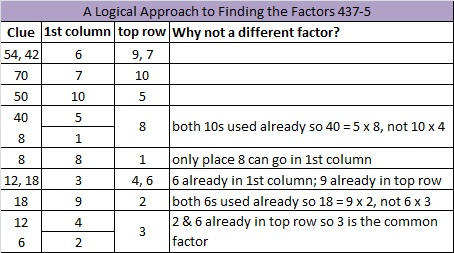# 236 and Level 5

• 236 is a composite number.
• Prime factorization: 236 = 2 x 2 x 59, which can be written (2^2) x 59
• The exponents in the prime factorization are 2 and 1. Adding one to each and multiplying we get (2 + 1)(1 + 1) = 3 x 2 = 6. Therefore 236 has 6 factors.
• Factors of 236: 1, 2, 4, 59, 118, 236
• Factor pairs: 236 = 1 x 236, 2 x 118, or 4 x 59
• Taking the factor pair with the largest square number factor, we get √236 = (√4)( √59) = 2√59 ≈ 15.362Print the puzzles or type the factors on this excel file: 10 Factors 2014-09-15